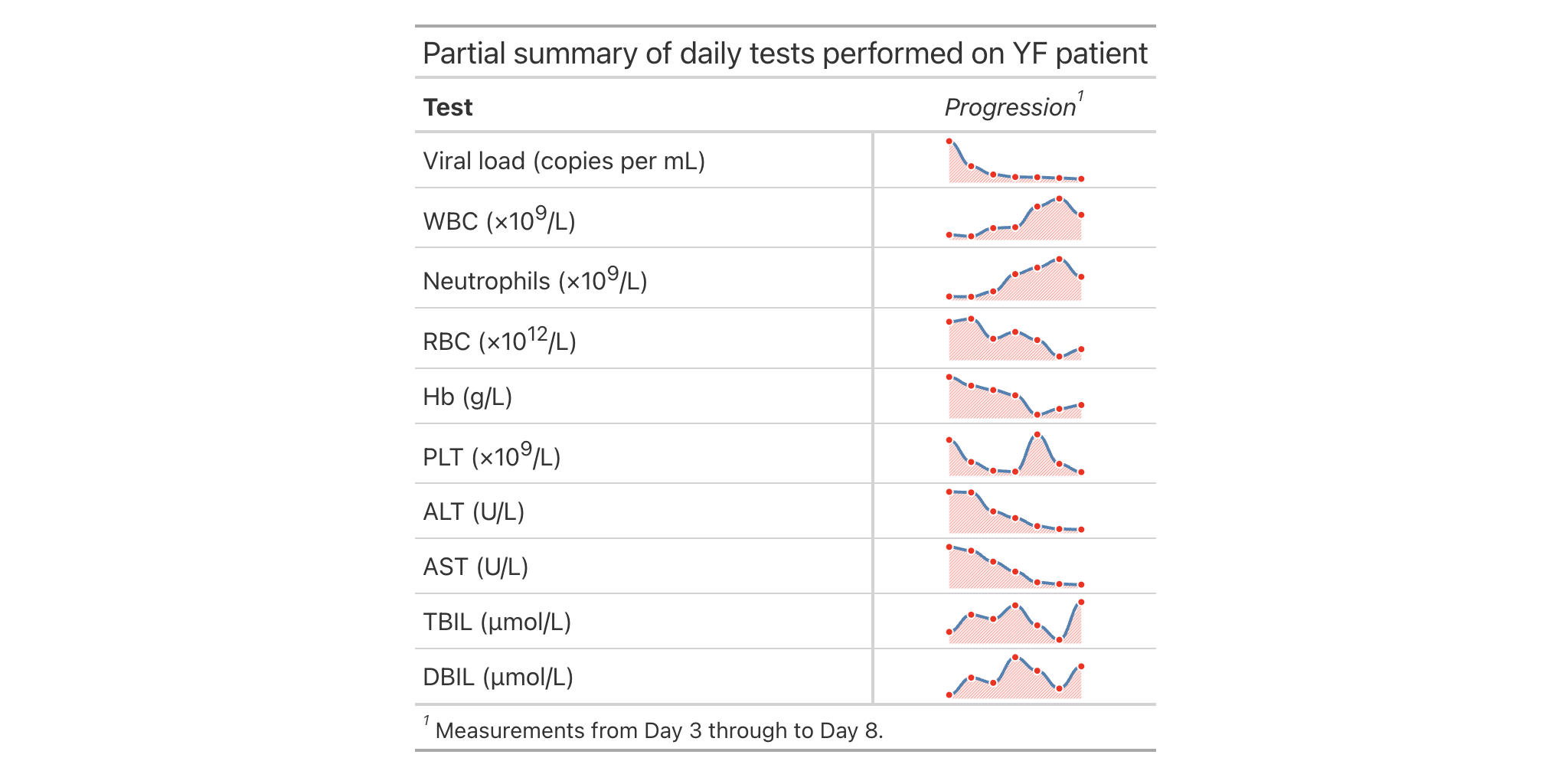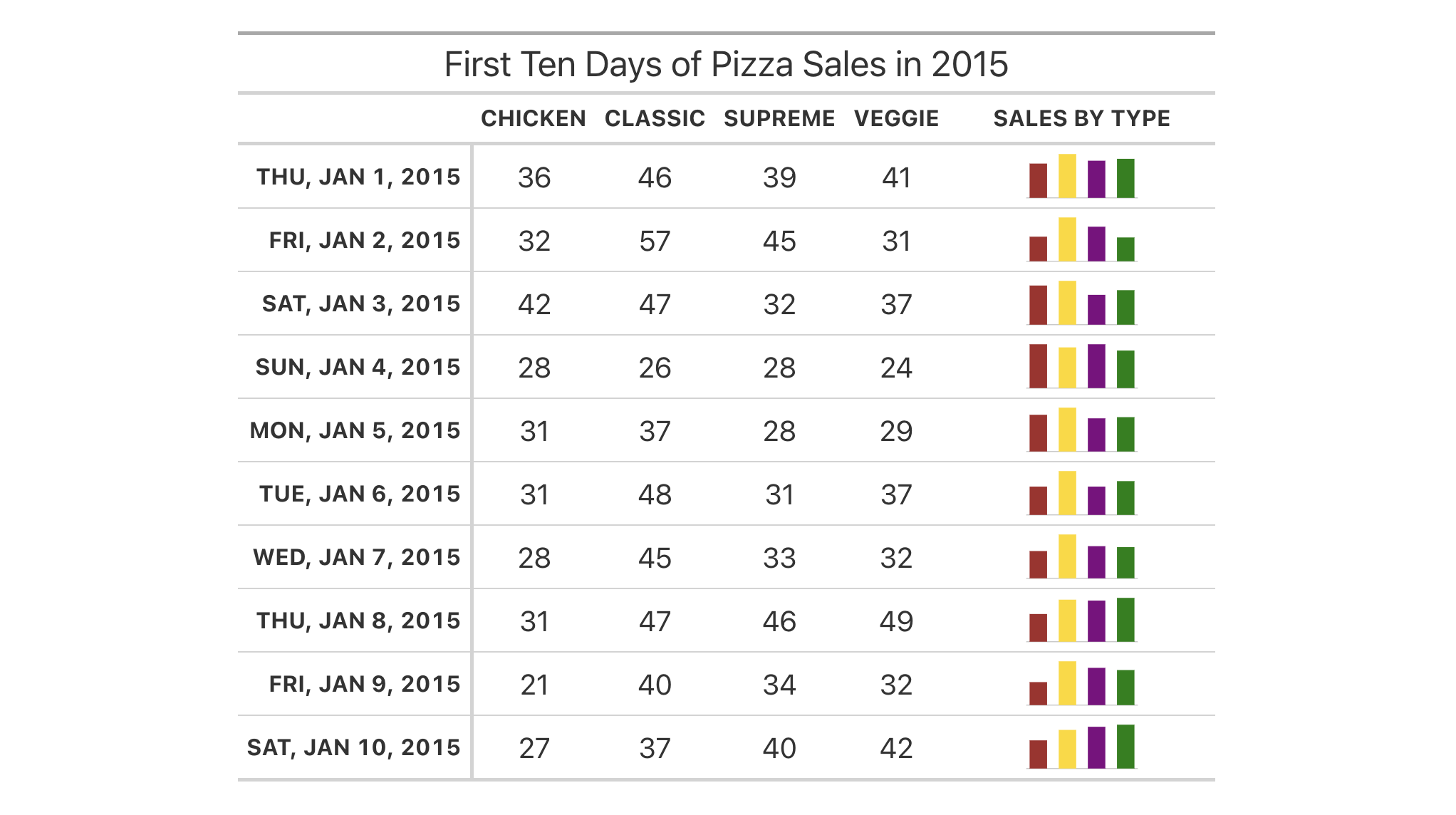Nanoplots are tiny plots you can use in your gt table. They are simple by design, mainly because there isn't a lot of space to work with. With that simplicity, however, you do get a set of very succinct data visualizations that adapt nicely to the amount of data you feed into them. With cols_nanoplot() you take data from one or more columns as the basic inputs for the nanoplots and generate a new column containing the plots.

Each nanoplot contains data points with reasonably good visibility, having smooth connecting lines between them to allow for easier scanning of values. By default, a nanoplot will have basic interactivity. One can hover over the data points and vertical guides will display values ascribed to each. A horizontal reference line is also present in the standard view (denoting the median of the data). This reference line can be customized by providing a static value or by choosing a keyword that computes a particular y value using a nanoplot's data values. Aside from a reference line, there is also an associated reference area which, by default, tries to make itself useful by bounding the area between the lower and upper quartiles of the data. These boundaries can also be customized in a similar fashion as the reference line. The nanoplots are robust against missing values, and multiple strategies are available for handling missingness.

While basic customization options are present in the cols_nanoplot(), many more opportunities for customizing nanoplots on a more granular level are possible with the nanoplot_options() helper function. That is to be invoked at the options argument of cols_nanoplot(). Through that helper function, layers of the nanoplots can be selectively removed and aesthetics of the remaining plot components can modified.

## Usage

cols_nanoplot(
data,
columns,
rows = everything(),
missing_vals = c("gap", "zero", "remove"),
reference_line = NULL,
reference_area = NULL,
currency = NULL,
new_col_name = NULL,
new_col_label = NULL,
before = NULL,
after = NULL,
height = NULL,
options = NULL
)

## Arguments

data

The gt table data object

obj:<gt_tbl> // required

This is the gt table object that is commonly created through use of the gt() function.

columns

Columns from which to obtain data

<column-targeting expression> // required

The columns which contain the numeric data to be plotted as nanoplots. Can either be a series of column names provided in c(), a vector of column indices, or a select helper function. Examples of select helper functions include starts_with(), ends_with(), contains(), matches(), one_of(), num_range(), and everything(). Data collected from the columns will be concatenated together in the order of resolution.

rows

Rows that should contain nanoplots

<row-targeting expression> // default: everything()

With rows we can specify which rows should contain nanoplots in the new column. The default everything() results in all rows in columns being formatted. Alternatively, we can supply a vector of row captions within c(), a vector of row indices, or a select helper function. Examples of select helper functions include starts_with(), ends_with(), contains(), matches(), one_of(), num_range(), and everything(). We can also use expressions to filter down to the rows we need (e.g., [colname_1] > 100 & [colname_2] < 50).

missing_vals

Treatment of missing values

singl-kw:[gap|zero|remove] // default: "gap"

If missing values are encountered within the input data, there are three strategies available for their handling: (1) "gap" will display data gaps at the sites of missing data, where data lines will have discontinuities; (2) "zero" will replace NA values with zero values; and (3) "remove" will remove any incoming NA values.

reference_line

scalar<numeric|integer|character> // default: NULL (optional)

Supplying a single value here will add a horizontal reference line. It could be a static numeric value, applied to all nanoplots generated. Or, the input can be one of the following for generating the line from the underlying data: (1) "mean", (2) "median", (3) "min", (4) "max", (5) "first", or (6) "last".

reference_area

vector<numeric|integer|character>|list // default: NULL (optional)

A reference area requires two inputs to define bottom and top boundaries for a rectangular area. The types of values supplied are the same as those expected for reference_line, which is either a static numeric value or one of the following keywords for the generation of the value: (1) "mean", (2) "median", (3) "min", (4) "max", (5) "first", or (6) "last". Input can either be a vector or list with two elements.

currency

Define values as currencies of a specific type

scalar<character>|obj:<gt_currency> // default: NULL (optional)

If the values are to be displayed as currency values, supply either: (1) a 3-letter currency code (e.g., "USD" for U.S. Dollars, "EUR" for the Euro currency), (2) a common currency name (e.g., "dollar", "pound", "yen", etc.), or (3) an invocation of the currency() helper function for specifying a custom currency (where the string could vary across output contexts). Use info_currencies() to get an information table with all of the valid currency codes, and examples of each, for the first two cases.

new_col_name

Column name for the new column containing the plots

scalar<character> // default: NULL (optional)

A single column name in quotation marks. Values will be extracted from this column and provided to compatible arguments. If not provided the new column name will be "nanoplots".

new_col_label

Column label for the new column containing the plots

scalar<character> // default: NULL (optional)

A single column label. If not supplied then the column label will inherit from new_col_name (if nothing provided to that argument, the label will be "nanoplots").

before, after

Column used as anchor

<column-targeting expression> // default: NULL (optional)

A single column-resolving expression or column index can be given to either before or after. The column specifies where the new column containing the nanoplots should be positioned among the existing columns in the input data table. While select helper functions such as starts_with() and ends_with() can be used for column targeting, it's recommended that a single column name or index be used. This is to ensure that exactly one column is provided to either of these arguments (otherwise, the function will be stopped). If nothing is provided for either argument then the new column will be placed at the end of the column series.

height

The height of the nanoplots

scalar<character> // default: NULL (optional)

The height of the nanoplots. If nothing is provided here then gt will provide a sensible length value of "1.5em".

options

Set options for the nanoplots

obj:<nanoplot_options // default: NULL (optional)

By using the nanoplot_options() helper function here, you can alter the layout and styling of the nanoplots in the new column.

## Value

An object of class gt_tbl.

## Targeting cells with columns and rows

Targeting of values to insert into the nanoplots is done through columns and additionally by rows (if nothing is provided for rows then entire columns are selected). Aside from declaring column names in c() (with bare column names or names in quotes) we can use also tidyselect-style expressions. This can be as basic as supplying a select helper like starts_with(), or, providing a more complex incantation like

where(~ is.numeric(.x) && max(.x, na.rm = TRUE) > 1E6)

which targets numeric columns that have a maximum value greater than 1,000,000 (excluding any NAs from consideration).

Once the columns are targeted, we may also target the rows within those columns. This can be done in a variety of ways. If a stub is present, then we potentially have row identifiers. Those can be used much like column names in the columns-targeting scenario. We can use simpler tidyselect-style expressions (the select helpers should work well here) and we can use quoted row identifiers in c(). It's also possible to use row indices (e.g., c(3, 5, 6)) though these index values must correspond to the row numbers of the input data (the indices won't necessarily match those of rearranged rows if row groups are present). One more type of expression is possible, an expression that takes column values (can involve any of the available columns in the table) and returns a logical vector.

## Examples

Let's make some nanoplots with the illness dataset. The columns beginning with 'day' all contain ordered measurement values, comprising seven individual daily results. Using cols_nanoplot() we create a new column to hold the nanoplots (with new_col_name = "nanoplots"), referencing the columns containing the data (with columns = starts_with("day")). It's also possible to define a column label here using the new_col_label argument.

illness |>
gt(rowname_col = "test") |>
tab_header("Partial summary of daily tests performed on YF patient") |>
cols_hide(columns = c(starts_with("norm"), starts_with("day"))) |>
fmt_units(columns = units) |>
cols_nanoplot(
columns = starts_with("day"),
new_col_name = "nanoplots",
new_col_label = md("*Progression*"),
options = nanoplot_options(
show_reference_line = FALSE,
show_reference_area = FALSE
)
) |>
cols_align(align = "center", columns = nanoplots) |>
cols_merge(columns = c(test, units), pattern = "{1} ({2})") |>
tab_footnote(
footnote = "Measurements from Day 3 through to Day 8.",
locations = cells_column_labels(columns = nanoplots)
)Now we'll make another table that contains two columns of nanoplots. Starting from the towny dataset, we first reduce it down to a subset of columns and rows. All of the columns related to either population or density will be used as input data for the two nanoplots. Both nanoplots will use a reference line that is generated from the median of the input data. And by naming the new nanoplot-laden columns in a similar manner as the input data columns, we can take advantage of select helpers (e.g., when using tab_spanner()). Many of the input data columns are now redundant because of the plots, so we'll elect to hide most of those with cols_hide().

towny |>
dplyr::select(name, starts_with("population"), starts_with("density")) |>
dplyr::filter(population_2021 > 200000) |>
dplyr::arrange(desc(population_2021)) |>
gt() |>
fmt_integer(columns = starts_with("population")) |>
fmt_number(columns = starts_with("density"), decimals = 1) |>
cols_nanoplot(
columns = starts_with("population"),
reference_line = "median",
reference_area = NA,
new_col_name = "population_plot",
new_col_label = md("*Change*")
) |>
cols_nanoplot(
columns = starts_with("density"),
reference_line = "median",
reference_area = NA,
new_col_name = "density_plot",
new_col_label = md("*Change*")
) |>
cols_hide(columns = matches("2001|2006|2011|2016")) |>
tab_spanner(
label = "Population",
columns = starts_with("population")
) |>
tab_spanner(
label = "Density ({{*persons* km^-2}})",
columns = starts_with("density")
) |>
cols_label_with(
columns = -matches("plot"),
fn = function(x) gsub("\\D+", "", x)
) |>
cols_align(align = "center", columns = matches("plot")) |>
cols_width(
name ~ px(140),
everything() ~ px(100)
) |>
opt_horizontal_padding(scale = 2)5-8

## Function Introduced

In Development

Other column modification functions: cols_add(), cols_align_decimal(), cols_align(), cols_hide(), cols_label_with(), cols_label(), cols_merge_n_pct(), cols_merge_range(), cols_merge_uncert(), cols_merge(), cols_move_to_end(), cols_move_to_start(), cols_move(), cols_unhide(), cols_units(), cols_width()